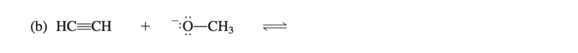Problem: Each of the following acid–base reactions involves substances found in Table 1.8. Use the pKa data in the table to help you predict the products of the reactions. Use curved arrows to show electron flow. Predict whether the equilibrium lies to the left or to the right and calculate the equilibrium constant for each reaction.

FREE Expert Solution
81% (280 ratings)
Problem Details

Each of the following acid–base reactions involves substances found in Table 1.8. Use the pKa data in the table to help you predict the products of the reactions. Use curved arrows to show electron flow. Predict whether the equilibrium lies to the left or to the right and calculate the equilibrium constant for each reaction.What scientific concept do you need to know in order to solve this problem?

Our tutors have indicated that to solve this problem you will need to apply the Acid Base Equilibrium concept. You can view video lessons to learn Acid Base Equilibrium Or if you need more Acid Base Equilibrium practice, you can also practice Acid Base Equilibrium practice problems .

What is the difficulty of this problem?

Our tutors rated the difficulty of Each of the following acid–base reactions involves substance... as low difficulty.

How long does this problem take to solve?

Our expert Organic tutor, Jonathan took less than a minute to solve this problem. You can follow their steps in the video explanation above.

What professor is this problem relevant for?

Based on our data, we think this problem is relevant for Professor Hollabaugh's class at UNG.

What textbook is this problem found in?

Our data indicates that this problem or a close variation was asked in . You can also practice practice problems .